19 products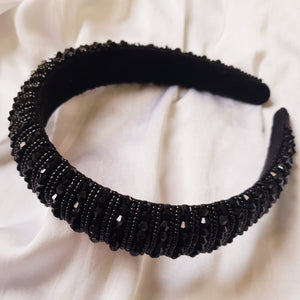Regular price
Rs. 849.00
Sale price
Rs. 849.00
Regular price
Rs. 1,199.00
Unit price
per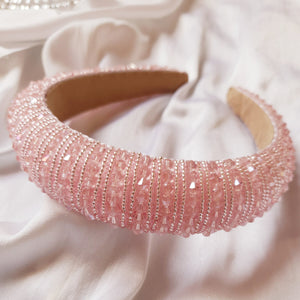Regular price
Rs. 849.00
Sale price
Rs. 849.00
Regular price
Rs. 1,199.00
Unit price
perRegular price
Rs. 499.00
Sale price
Rs. 499.00
Regular price
Rs. 699.00
Unit price
per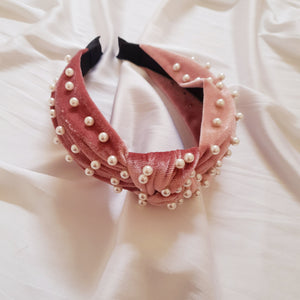Regular price
Rs. 499.00
Sale price
Rs. 499.00
Regular price
Rs. 699.00
Unit price
perRegular price
Rs. 499.00
Sale price
Rs. 499.00
Regular price
Rs. 699.00
Unit price
perRegular price
Rs. 349.00
Sale price
Rs. 349.00
Regular price
Rs. 499.00
Unit price
perRegular price
Rs. 349.00
Sale price
Rs. 349.00
Regular price
Rs. 499.00
Unit price
perRegular price
Rs. 349.00
Sale price
Rs. 349.00
Regular price
Rs. 499.00
Unit price
per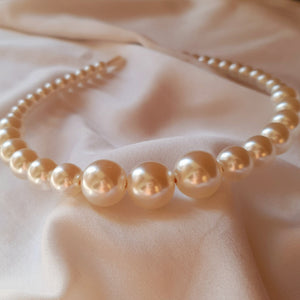Regular price
Rs. 299.00
Sale price
Rs. 299.00
Regular price
Rs. 349.00
Unit price
perRegular price
Rs. 299.00
Sale price
Rs. 299.00
Regular price
Rs. 349.00
Unit price
perRegular price
Rs. 349.00
Sale price
Rs. 349.00
Regular price
Rs. 449.00
Unit price
perRegular price
Rs. 349.00
Sale price
Rs. 349.00
Regular price
Rs. 449.00
Unit price
perRegular price
Rs. 349.00
Sale price
Rs. 349.00
Regular price
Rs. 449.00
Unit price
perRegular price
Rs. 299.00
Sale price
Rs. 299.00
Regular price
Rs. 349.00
Unit price
perRegular price
Rs. 299.00
Sale price
Rs. 299.00
Regular price
Rs. 349.00
Unit price
per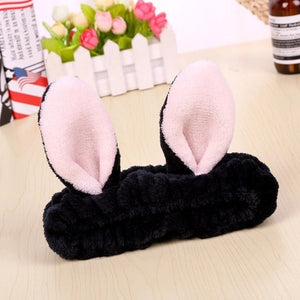Regular price
Rs. 249.00
Sale price
Rs. 249.00
Regular price
Rs. 299.00
Unit price
perRegular price
Rs. 299.00
Sale price
Rs. 299.00
Regular price
Rs. 349.00
Unit price
per
• Striped Turbanknot HairbandRegular price
Rs. 169.00
Sale price
Rs. 169.00
Regular price
Rs. 199.00
Unit price
per